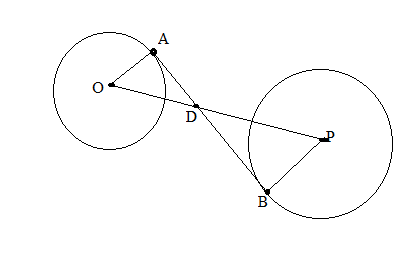Chapter 6.3, Problem 39E### Elementary Geometry for College St...

6th Edition
Daniel C. Alexander + 1 other
ISBN: 9781285195698

#### Solutions

Chapter
Section### Elementary Geometry for College St...

6th Edition
Daniel C. Alexander + 1 other
ISBN: 9781285195698
Textbook Problem
1 views

# The center of a circle of radius 2 inches is at a distance of 10 inches from the center of a circle of radius length 3 inches. To the nearest tenth of an inch, what is the approximate length of a common internal tangent? Use the hint provided in Exercise 38.(HINT: use similar triangles to find OD and DP. Then apply the Pythagorean Theorem twice.)To determine

To find:

To find length of a common internal tangent.

Explanation

Given that, the center of a circle of radius 2 in. is at a distance of 10 in. from the center of a circle of radius length 3 in.

That is OA¯=2,PB¯=3 and OP¯=10 then AB¯ is common internal tangent.

The diagrammatic representation is given below,

Since AB¯ is common internal tangent therefore, AB¯OA¯ and AB¯PB¯

Using the vertical angle theorem to get the following,

If two triangles are similar, then the ration of any two corresponding segments (such as altitudes, medians, or angle bisectors) equals the ratio of any two corresponding sides.

OA¯PB¯=OD¯PD¯23=OD¯PD¯OD¯PD¯=233OD¯=2PD¯

We know that,

OP¯=OD¯+PD¯10=OD¯+PD¯PD¯=10OD¯. Since OP¯=10

Substitute this in the above equation to get the following,

### Still sussing out bartleby?

Check out a sample textbook solution.

See a sample solution

#### The Solution to Your Study Problems

Bartleby provides explanations to thousands of textbook problems written by our experts, many with advanced degrees!

Get Started

#### In Exercises 1124, find the indicated limits, if they exist. 15. limx2x+3x29

Applied Calculus for the Managerial, Life, and Social Sciences: A Brief Approach

#### Evaluate the integrals in Problems 1-32. 29.

Mathematical Applications for the Management, Life, and Social Sciences

#### True or False: converges conditionally.

Study Guide for Stewart's Single Variable Calculus: Early Transcendentals, 8th